# Systems Of Linear Equations With No Solution (3 Ways To Tell)

When working with systems of linear equations, we often see one or infinitely many solutions.  However, it is also possible that a linear system will have no solution.

So, when does a system of linear equations have no solution?  A system of two linear equations in two variables has no solution when the two lines are parallel. From an algebra standpoint, this means that we get a false equation when solving the system.  Visually, the lines never intersect on a graph, since they have the same slope but different y-intercepts.

Of course, a system of three equations in three variables has no solution if there is no point where all three planes intersect.

In this article, we’ll talk about how you can tell that a system of linear equations has no solution.  We’ll also look at some examples of linear systems with no solution in 2 variables and in 3 variables.

Let’s begin.

Having math trouble?

Looking for a tutor?

## Systems Of Linear Equations With No Solution

A system of linear equations can have no solution if the equations are inconsistent.  This means that there is no point that can satisfy all of the equations at the same time.

The image below summarizes the 3 possible cases for the solutions for a system of 2 linear equations in 2 variables.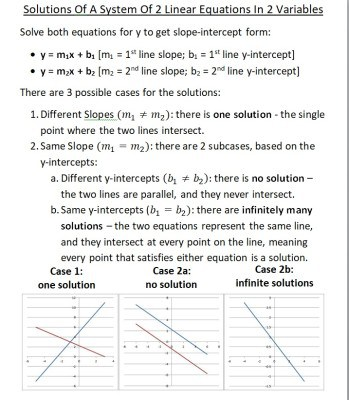A system of 2 linear equations in 2 variables has no solution when the two lines have the same slope and different y-intercepts (that is, they are parallel and never intersect).

A system of equations in 2, 3, or more variables can have no solution.  We’ll start with linear equations in 2 variables with no solution.

## When Does A Linear System Have No Solution? (System Of Linear Equations In 2 Variables)

There are a few ways to tell when a linear system in two variables has no solution:

We’ll look at some examples of each case, starting with solving the system.

### Solving A Linear System With No Solution

When we attempt to solve a linear system with no solution, we will get a nonsense equation as a result.  For example, after we simplify and combine like terms, we will get something like 0 = 1 or 2 = 5.

Let’s take a look at some examples to see how this can happen.

#### Example 1: Using Elimination To Show A Linear System Has No Solution

Let’s say we want to solve the following system of linear equations:

• 3x + 4y = 9
• -6x – 8y = 10

We will use elimination to solve.  Let’s try to eliminate the “x” variable.

We begin by multiplying the first equation by 2 to get:

• 2(3x + 4y) = 2(9)  [multiply the first equation by 2 on both sides]
• 6x + 8y = 18  [distribute the 2 through parentheses]

Now we add this modified equation to the second one:

6x + 8y = 18

+

-6x – 8y = 10

___________

0x – 0y = 28

0 = 28

Since this equation is nonsense (it can never be true), this tells us that there is no solution to this system of linear equations. The graph below confirms that the lines are parallel and have no solution (they never intersect).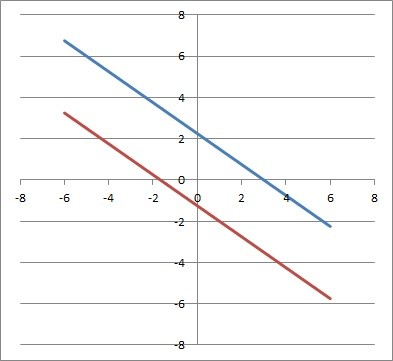The two lines 3x + 4y = 9 (blue) and -6x – 8y = 10 (red) are parallel, so they never intersect, and there is no solution to the linear system.

#### Example 2: Using Substitution To Show A Linear System Has No Solution

Let’s say we want to solve the following system of linear equations:

• y = 2x + 5
• y = 2x + 8

We will use substitution to solve.  We’ll substitute the y from the first equation into the y in the second equation:

• 2x + 5 = 2x + 8  [substitute y = 2x + 5 from the first equation]
• 2x – 2x = 8 – 5  [put like terms on both sides]
• 0x = 3  [combine like terms]
• 0 = 3

Since this equation is nonsense (it can never be true), this tells us that there is no solution to this system of linear equations. The graph below confirms that the lines are parallel and have no solution (they never intersect).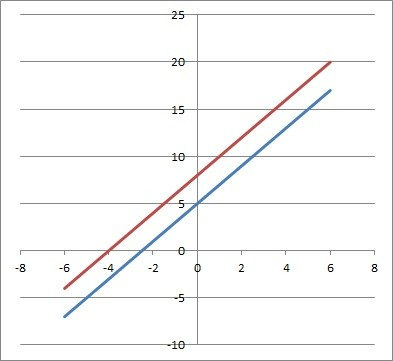The two lines y = 2x + 5 (blue) and y = 2x + 8 (red) are parallel, so they never intersect, and there is no solution to the linear system.

### Looking At The Graph Of A Linear System With No Solution

When we graph a linear system with no solution, we will get two parallel lines that never intersect.

Let’s take a look at some examples to see how this can happen.

#### Example 1: Graph Of Two Parallel Lines From A Linear System With No Solution

Let’s graph the following system of linear equations:

• y = -3x + 4
• y = -3x – 2

Both lines will have a slope of -3, but they have different y-intercepts, as you can see in the graph below: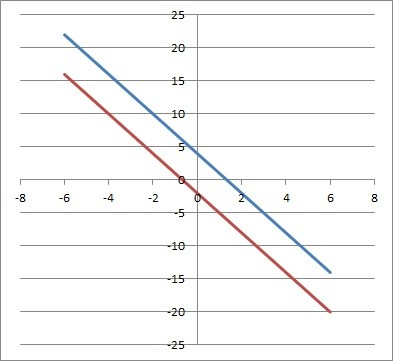The two lines y = -3x + 4 (blue) and y = -3x – 2 (red) are parallel, so they never intersect, and there is no solution to the linear system.

Since the lines are parallel, they will never intersect.  This means that there is no solution to the linear system we started with (there is no point that lies on both lines).

#### Example 2: Graph Of Two Parallel Vertical Lines From A Linear System With No Solution

Let’s graph the following system of linear equations:

• y = 5
• y = -3

Both lines will have a slope of 0, but they have different y-intercepts, as you can see in the graph below: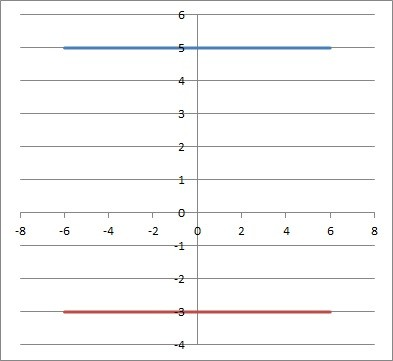The two lines y = 5 (blue) and y = -3 (red) are parallel, so they never intersect, and there is no solution to the linear system.

Since the lines are parallel, they will never intersect.  This means that there is no solution to the linear system we started with (there is no point that lies on both lines).

#### Example 3: Graph Of Two Parallel Horizontal Lines From A Linear System With No Solution

Let’s graph the following system of linear equations:

• x = 2
• x = -4

Both lines will have an undefined slope, since they are vertical lines.  They also have no y-intercept, but they are parallel, as you can see in the graph below: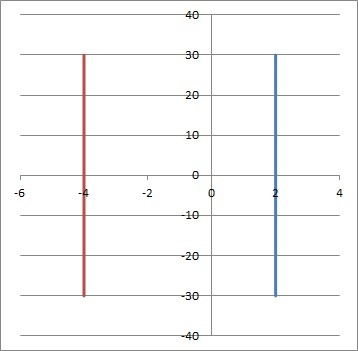The two lines x = 2 (blue) and x = -4 (red) are parallel, so they never intersect, and there is no solution to the linear system.

Since the lines are parallel, they will never intersect.  This means that there is no solution to the linear system we started with (there is no point that lies on both lines).

Having math trouble?

Looking for a tutor?

### Looking At The Slope & Y-Intercept Of A Linear System With No Solution

When we solve a linear equation for y, we get slope-intercept form.  If we do this for both equations in a linear system, we can compare the slope and y-intercept.

If the two slopes are the same and the y-intercepts are different, then the two lines are parallel, meaning they never intersect and there is no solution to the linear system.

Let’s take a look at some examples to see how this can happen.

#### Example 1: Comparing Slope & Y-Intercept To Show There Is No Solution To A System Of Two Linear Equations

Let’s say we have the following system of linear equations:

• 4x = – 2y + 8
• 3y = -6x + 9

We will solve for y in both equations to get slope-intercept form, y = mx + b.

Solving the first equation for y, we get:

• 4x = – 2y + 8
• 4x + 2y = 8  [add 2y to both sides]
• 2y = -4x + 8  [subtract 4x from both sides]
• y = -2x + 4  [divide by 2 on both sides]

Solving the second equation for y, we get:

• 3y = -6x + 9
• y = -2x + 3  [divide by 3 on both sides]

So, the two equations in slope-intercept form are:

• y = -2x + 4
• y = -2x + 3

Since these two equations have the same slope (m = -2) and different y-intercepts (4 and 3), we know that the two lines are parallel.  Since the lines never intersect, there is no solution to the system (no point that is on both lines).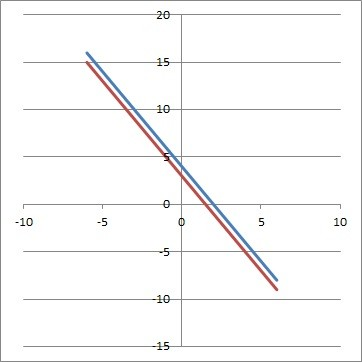The two lines y = -2x + 4 (blue) and y = -2x + 3 (red) are parallel, so they never intersect, and there is no solution to the linear system.

#### Example 2: Comparing Slope & Y-Intercept To Show There Is No Solution To A System Of Two Linear Equations

Let’s say we have the following system of linear equations:

• 40x = 5y – 15
• 4y – 32x + 5 = 13

We will solve for y in both equations to get slope-intercept form, y = mx + b.

Solving the first equation for y, we get:

• 40x = 5y – 15
• 40x + 15 = 5y  [add 15 to both sides]
• 8x + 3 = y  [divide by 5 on both sides]

Solving the second equation for y, we get:

• 4y – 32x + 5 = 13
• 4y – 32x = 13 – 5  [subtract 5 from both sides]
• 4y – 32x = 8  [combine like terms: constants]
• 4y = 32x + 8  [add 32x to both sides]
• y = 8x + 2  [divide by 4 on both sides]

So, the two equations in slope-intercept form are:

• y = 8x + 3
• y = 8x + 2

Since these two equations have the same slope (m = 8) and different y-intercepts (3 and 2), we know that the two lines are parallel.  Since the lines never intersect, there is no solution to the system (no point that is on both lines).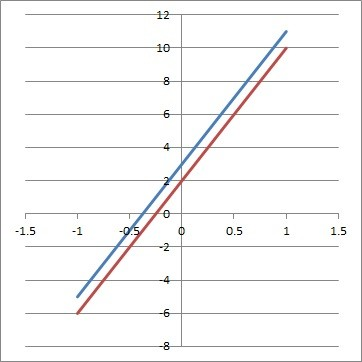The two lines y = 8x + 3 (blue) and y = 8x + 2 (red) are parallel, so they never intersect, and there is no solution to the linear system.

## How To Create A System Of Linear Equations With No Solution

To create a system of linear equations with no solution, we can take a couple of approaches:

• One method is to start with a “nonsense” equation and add variables until we get two linear expressions, one on each side of the equation.
• Another method is to write two lines in slope-intercept form y = mx + b, where the slopes are the same and the y-intercepts are different.

Let’s try each method in turn.

### Example 1: Create A System Of Linear Equations With No Solution

• 0 = 1

Now we’ll add x to both sides:

• x + 0 = x + 1
• x = x + 1

Next we’ll multiply by 3 on both sides:

• 3x = 3x + 3

Now we have a linear expression on each side of the equation, and we can use these two give us the equations of two lines that never intersect:

• y = 3x  [from the left side of the equation above]
• y = 3x + 3  [from the right side of the equation above]

You can see that these lines are parallel from the graph below, meaning there is no solution to the system.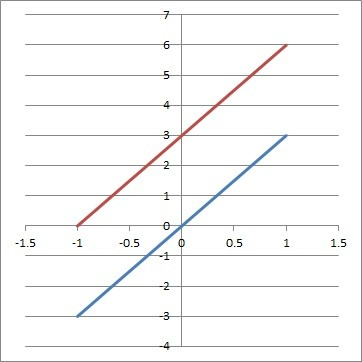The two lines y = 3x (blue) and y = 3x + 3 (red) are parallel, so they never intersect, and there is no solution to the linear system.

### Example 2: Create A System Of Linear Equations With No Solution

Let’s write the equations of two lines in slope-intercept form (that is, y = mx + b) with the same slope and different y-intercepts.

We’ll use a common slope of 2, with y-intercepts of 5 and 7:

• y = 2x + 5  [slope of 2, y-intercept of 5]
• y = 2x + 7  [slope of 2, y-intercept of 7]

You can see that these lines are parallel from the graph below, meaning there is no solution to the system.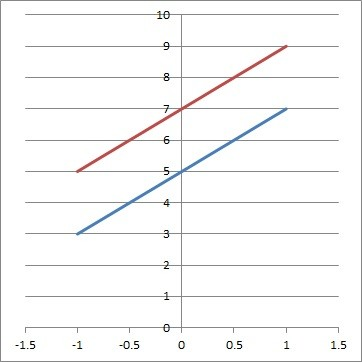The two lines y = 2x + 5 (blue) and y = 2x + 7 (red) are parallel, so they never intersect, and there is no solution to the linear system.

## System Of Linear Equations In Three Variables With No Solution

A system of equations in 3 variables will have no solution if there is no point where the 3 planes all intersect.  This can happen if:

• The planes are all “parallel” (they never intersect one another at all).
• Two planes may intersect, but never all three at once.

Here is an example of the first case:

• x + y + z = 1
• x + y + z = 2
• x + y + z = 3

Note that all of these plane equations have the same expression on the left side, but different constants on the right side.

In the same way that x = 1 and x = 2 cannot be true at the same time, we can also say that x + y + z = 1 and x + y + z = 2 cannot be true at the same time.

This means that there is no solution to the above system.

## When Does A System Of Linear Equations Have A Solution?

A system of linear equations in two variables has a solution when the two lines intersect in at least one place.

• If the two lines have the same slope and the same y-intercept, then the two equations are equivalent, and they represent the same line (so there are infinitely many solutions, since every point on the line is a solution).
• If the two lines have different slopes, then they intersect at exactly one point.

## Conclusion

Now you know when a system of linear equations has no solution.  You also know what to look out for in terms of the slope, y-intercept, and graph of lines in these systems.

You might also find it helpful to read my article on systems of linear equations with infinite solutions.